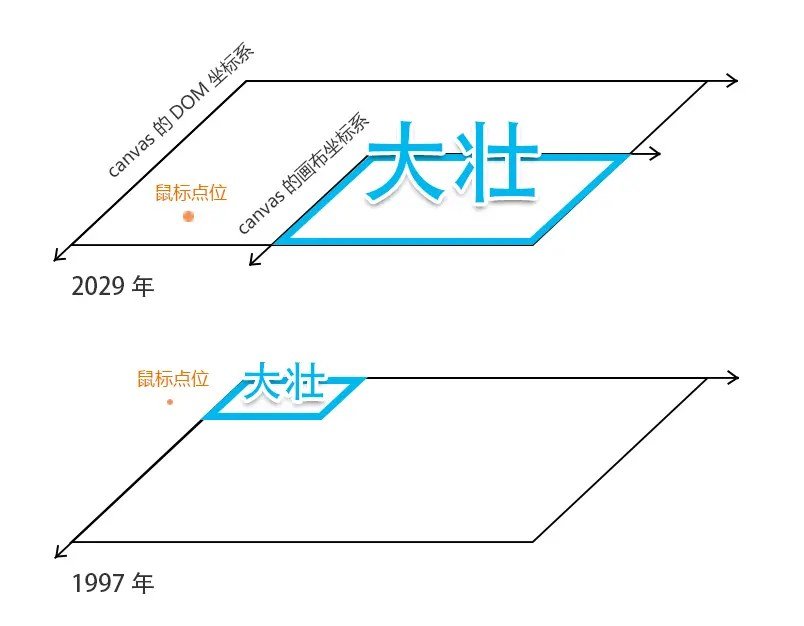# Graphic selection-material is not easy

Posted May 26, 20203 min read

In this chapter, let's talk about how the mouse selects the transformed graphics.
First of all, let me give you a chestnut:At the end of the war in 2029, the Terminator wanted to kill the human leader, but the strength is too strong, and its strength requires complex operations to know. Therefore, the Terminator wanted to go back to 1997 and kill it under the known conditions of being strong and weak. In this way, according to the law of material difficulty, the human leader in the battle of the end times in 2029 will not exist.

Next, let's decipher the method of the Terminator's shuttle time.

#### Terminator needs to know the data:

• The initial attributes of Zhuang in 1997, such as the set of vertices that make up the Zhuang silhouette;
• Transformation information from 1997 to 2029, such as how much it moves, how much it rotates, and how much it grows.

According to the law of material difficulty:material does not change, space does not change; space does not change, time does not change.
It is still true to reverse the laws that matter is not easy:matter changes, space changes; space changes, time changes.
So the Terminator wants to go back to 1997, as long as he reversely changes his position according to the strong transformation rules, he can go back to 1997.

#### For example:

• From 1997 to 2029, Zhuang moved 100 along the x-axis and 200 along the y-axis, rotated 90 degrees, and doubled.
• The terminator(mouse point) will move -100 along the x-axis, -200 along the y-axis, and rotate -90 degrees, the distance between the point and the center point will be reduced by 2 times

Note:The transformation sequence of the terminator must be the same as the transformation sequence of the strong; the change of the terminator is only the point, the point has no size, and the point transformation is essentially the displacement of the coordinate system of the canvas where the target object is located. Only in this way, when the Terminator shuttled to 1997, can it be accurately positioned and strong.
Icon:Next, we will walk through this principle in the code:
Draw a love first, the canvas coordinate system on which the canvas is located has been displaced in the x and y directions(300,400)

``````const poly = new Poly({
position:new Vector2(300,400),
stroke:true,
close:true,
crtPath:function(ctx) {
ctx.beginPath();
ctx.moveTo(0,0);
ctx.bezierCurveTo(-200, -50, -180, -300,0, -200);
ctx.bezierCurveTo(180, -300,200, -50,0,0);
}
});
poly.draw(ctx);``````If my mouse wants to select this love, its position will be reversely transformed based on the love's transformation information:the x and y directions are shifted(-300, -400) respectively. code show as below:

``````const mousePos = getMousePos(event);
poly.crtPath(ctx);
const [nx, ny]= [
mousePos.x-poly.position.x,
mousePos.y-poly.position.y``````

];
const bool = ctx.isPointInPath(nx, ny);

After the displacement in the graphics transformation is over, the same applies to its rotation and scaling, which is to reverse the position of the mouse based on the transformation information of the graphics.
Below I directly encapsulate all the transformation methods into the method of obtaining the mouse point, that is getMousePos(event, poly), event is an event, poly is a graphic.
code show as below:

``````const getMousePos = function(event, obj = null) {
//Get the mouse position
const {clientX, clientY} = event;
//Get the border position of canvas
const {top, left} = canvas.getBoundingClientRect();
//Calculate the position of the mouse in the canvas
const x = clientX-left;
const y = clientY-top;
const mousePos = new Vector2(x, y);
if(obj) {
const {position, scale, rotation} = obj;
mousePos.sub(position);
mousePos.rotate(-rotation);
mousePos.divide(scale);
}
return mousePos;
};``````

mousePos is a Vector2 object, which encapsulates common methods about vectors. Such as:

``````export default class Vector2 {
constructor(x = 0, y = 0) {
this.x = x;
this.y = y;
}
//Subtraction
sub(v) {
this.x-= v.x;
this.y-= v.y;
return this;
}
//rotate based on origin
rotate(angle) {
const c = Math.cos(angle), s = Math.sin(angle);
const {x, y} = this;
this.x = x * c-y * s;
this.y = x * s + y * c;
return this;
}
//Vector division
divide(v) {
this.x/= v.x;
this.y/= v.y;
return this;
}
//...
}``````

Well, we talked about the selection of the transformed graphics.
In fact, there are many methods for selecting graphics and graphics. In the next chapter, I will tell you a method for selecting graphics:[Graphic Selection-Grid Selection]

Note:The material is not easy to read from the class of Xiuxian novels, there is no scientific basis, just to help everyone understand the code.